# Overview

We will learn how to use the package MAST to analyze single cell RNAseq experiments.

Starting from a matrix of counts of transcripts (cells by transcripts), we will discuss the preliminary steps of quality control, filtering, and exploratory data analysis. Once we are satisfied that we have high-quality expression, we will consider tests for differential expression and ways to visualize results. It is often helpful to synthesize from gene-level into module-level statements. Therefore, we will learn how to use MAST to test for gene set enrichment.

We will apply these methods to a data set of Mucosal Associated Invariant T cells (MAITs), measured on the Fluidigm C1. Half of the cells have been cytokine stimulated.

library(devtools)
library(BiocInstaller)
useDevel()
biocLite('SummarizedExperiment')
biocValid()
install_github('RGLab/MAST@summarizedExpt')

If you are running this at BioC, you should not need to run this chunk. Otherwise you will need to install the summarizedExpt branch and the devel (3.4) branch of Bioconductor.

suppressPackageStartupMessages({
library(ggplot2)
library(GGally)
library(GSEABase)
library(limma)
library(reshape2)
library(data.table)
library(knitr)
library(TxDb.Hsapiens.UCSC.hg19.knownGene)
library(stringr)
library(NMF)
library(rsvd)
library(RColorBrewer)
library(MAST)
})
#options(mc.cores = detectCores() - 1) #if you have multiple cores to spin
options(mc.cores = 1)
knitr::opts_chunk$set(message = FALSE,error = FALSE,warning = FALSE,cache = TRUE,fig.width=8,fig.height=6, auto.dep=TRUE) # Loading and transforming data freq_expressed <- 0.2 FCTHRESHOLD <- log2(1.5) First, let’s set some constants that we can use throughout this analysis. data(maits, package='MAST') dim(maits$expressionmat)
head(maits$cdat) head(maits$fdat)

Next, let’s load the data, which consists of a matrix of log2 + 1 transcripts per million (TPM), as output by RSEM. Internally, we use the packages RNASeqPipelineR and preprocessData to facilitate aligning and quantitating the raw reads. This is an experiment on Mucosal Associated Invariant T-cells from a single healthy donor. A number of cells were subjected to reverse transcription and library prep immediately, while others were first stimulated for 24 hours with a cytokine cocktail.

scaRaw <- FromMatrix(t(maits$expressionmat), maits$cdat, maits$fdat) FromMatrix constructs an object from a matrix (genes $$\times$$ cells) of expression, a data.frame of cell-level covariance and a data.frame of feature-level covariates. In this case, there are 96 single cells measured over 16302 genes. We derive from the SummarizedExperiment class, which makes it easy for feature (row) and cell (column) metadata to come along for the ride. There is also a constructor, FromFlatDF for flattened data where measurements, and cell and feature covariates are intermingled. ## Exploratory Data Analysis Let’s explore these data with a heatmap and some PCA. aheatmap(assay(scaRaw[1:1000,]), labRow='', annCol=as.data.frame(colData(scaRaw)[,c('condition', 'ourfilter')]), distfun='spearman')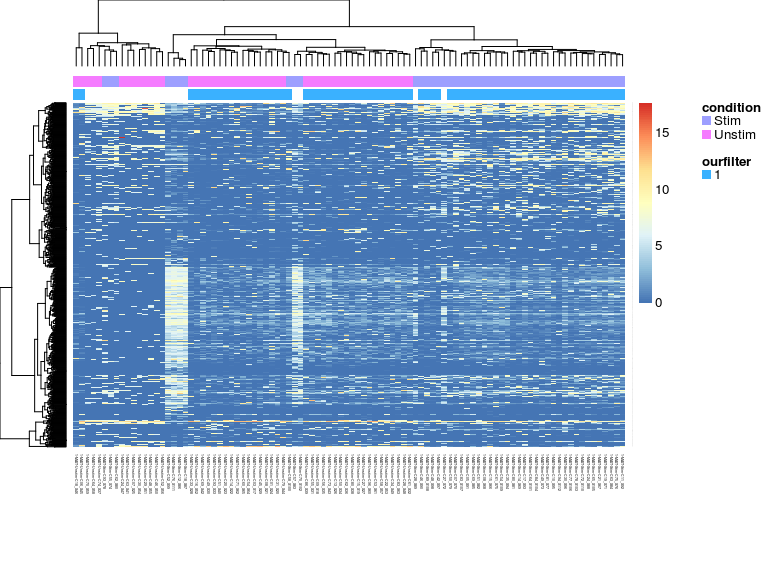set.seed(123) plotPCA <- function(sca_obj){ projection <- rpca(t(assay(sca_obj)), retx=TRUE, k=4)$x
pca <- data.table(projection,  as.data.frame(colData(sca_obj)))
print(ggpairs(pca, columns=c('PC1', 'PC2', 'PC3', 'libSize', 'PercentToHuman', 'nGeneOn', 'exonRate'),
mapping=aes(color=condition), upper=list(continuous='blank')))
invisible(pca)
}

plotPCA(scaRaw)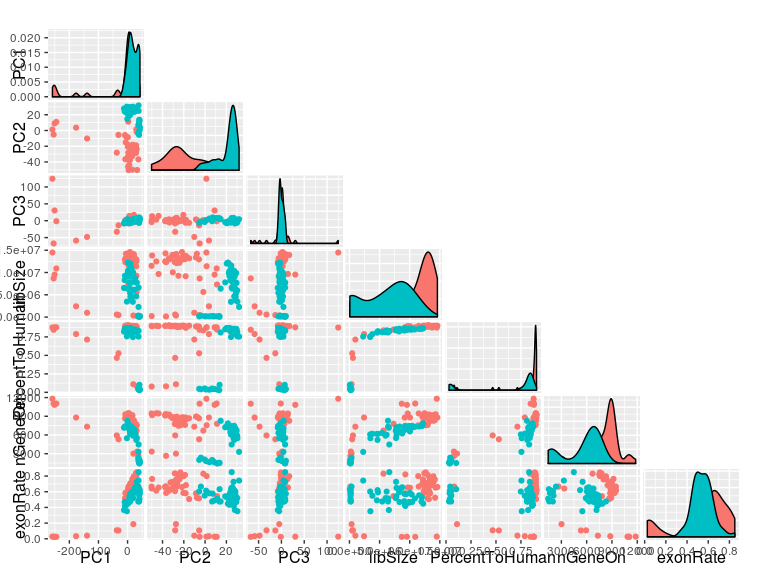filterCrit <- with(colData(scaRaw), pastFastqc=="PASS"& exonRate >0.3 & PercentToHuman>0.6 & nGeneOn> 4000)

From the PCA and heatmap we can tell that we’ve got some data quality issues with low-diversity libraries, and libraries where we capture a lot of non mRNA. We’ll set a filtering threshold using the Potter Stewart method, though Shalek et al and others have come up with more principled ways, such as training SVM on the expression data and quality metrics to predict failed libraries. Though this still presupposes a set of labeled “failures” and “successes”.

## Filtering

sca <- subset(scaRaw,filterCrit)
eid <- select(TxDb.Hsapiens.UCSC.hg19.knownGene,keys = mcols(sca)$entrez,keytype ="GENEID",columns = c("GENEID","TXNAME")) ueid <- unique(na.omit(eid)$GENEID)
sca <- sca[mcols(sca)$entrez %in% ueid,] ## Remove invariant genes sca <- sca[freq(sca)>0,] We’ll now consider only cells that pass the filter. subset(scaRaw, filterCrit) is just syntactic sugar for subsetting by columns, but who doesn’t like a little sugar? We’ll also limit ourselves to transcripts that have an EntrezGene id to facilitate interpretation. ### Recalculating the cellular detection rate (ngeneson) We and others have found that the number of genes detected in a sample, which we deemed the cellular detection rate is often the first principal component. After we removed genes low expression, perhaps we want to recalculate it. cdr2 <-colSums(assay(sca)>0) qplot(x=cdr2, y=colData(sca)$nGeneOn) + xlab('New CDR') + ylab('Old CDR')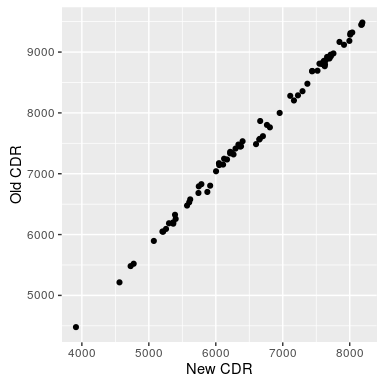We can assign this to a new column in the colData in the obvious fashion:

colData(sca)$cngeneson <- scale(cdr2) ### PCA on filtered cells plotPCA(sca)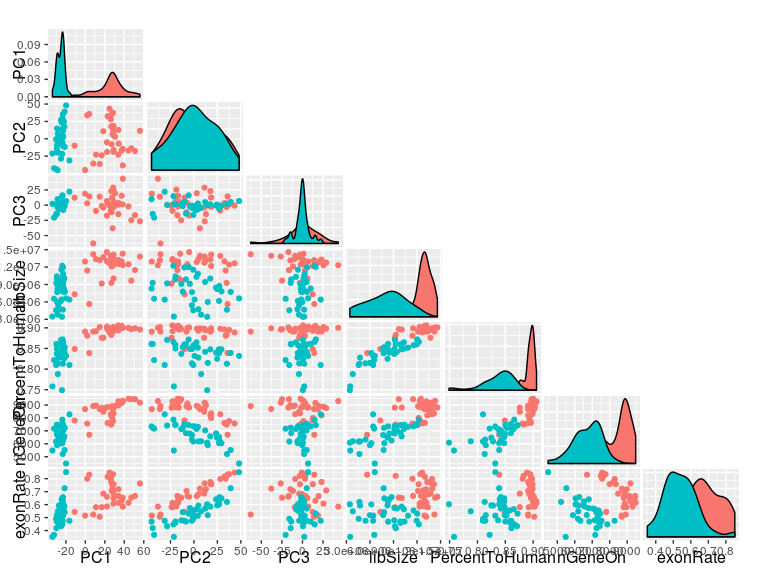PC1 now primarily captures stimulation effects. We still observe that PC2 correlates with the exonRate and ngeneson, but the data are more smoothly distributed. ### Exercises on loading and transforming data 1. These samples were reverse-transcribed and amplified on two Fluidigm C1 chips (and stimulation is completely confounded with chip…) The chip location is contained in the column wellKey in colData(sca) as a three-character string like CXX. How could you extract the chip location and add it as column to the cellular data? Hint: str_split_fixed or str_extract may be your friends here. # Adaptive thresholding Single cell gene expression data are known to be zero-inflated and bimodal, which is feature we observe here as well. scaSample <- sca[sample(which(freq(sca)>.1), 20),] flat <- as(scaSample, 'data.table') ggplot(flat, aes(x=value))+geom_density() +facet_wrap(~symbolid, scale='free_y')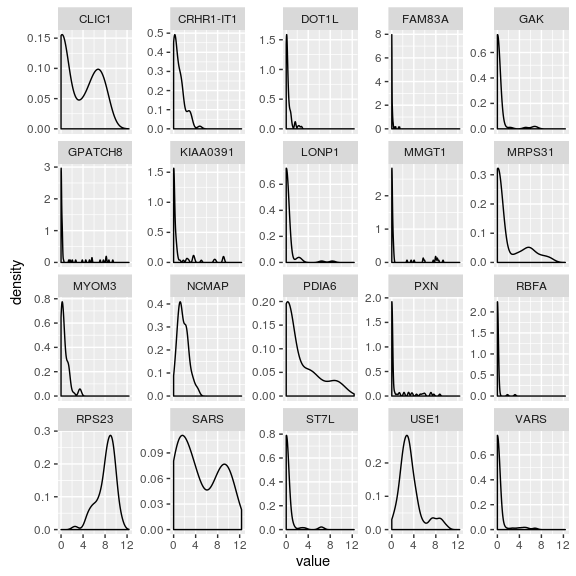Here we’ve taken subsample of size 20, then flattened it into a data.table to make it easy to plot with ggplot2. thres <- thresholdSCRNACountMatrix(assay(sca), nbins = 20, min_per_bin = 30) par(mfrow=c(5,4)) plot(thres)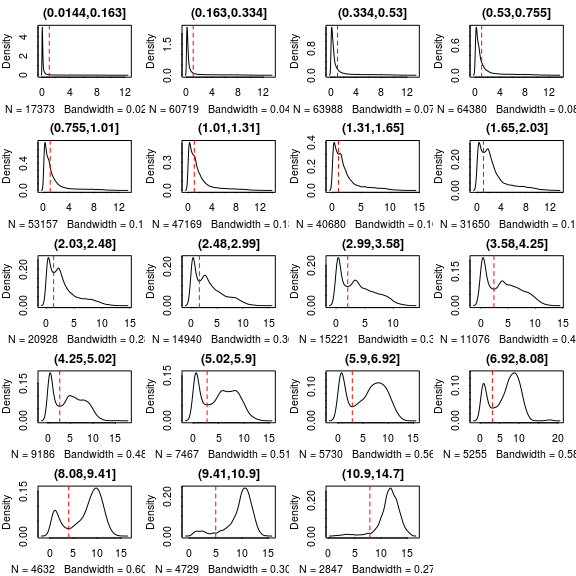We suspect that the left-most mode corresponds to non-specific hybridization of mRNA or genomic DNA. Here we apply an adaptive scheme to threshold values below a cut-off that depends on the intensity of the signal cluster from the gene (determined from the median expression value). When we plot the threshold vs genes binned by median expression value, we can see this evolution of thresholding value. assays(sca) <- list(thresh=thres$counts_threshold, tpm=assay(sca))
expressed_genes <- freq(sca) > freq_expressed
sca <- sca[expressed_genes,]

We’ll limit ourselves to genes that are found in at least 0.2 of the sample (since we won’t have any power to conclude much about less frequent transcripts).

### Exercises on thresholding

1. Many modeling procedures assume (or perform best) when the data are Normally distributed. How could we evaluate how thresholding affects the Normality of the data? How about the Normality of the non-zero data?

# Differential Expression using a Hurdle model

We’ll fit a hurdle model, modeling the condition and (centered) ngeneson factor, thus adjusting for the cellular detection rate.

In order to have more interpretable coefficients, we’ll set the reference level of the factor to be the “unstimulated” cells.

cond<-factor(colData(sca)$condition) cond<-relevel(cond,"Unstim") colData(sca)$condition<-cond
zlmCond <- zlm.SingleCellAssay(~condition + cngeneson, sca)
# The following are equivalent
## lrt <- lrTest(zlm, "condition")
## lrt <- lrTest(zlm, CoefficientHypothesis('conditionStim'))

# This would test if 2*cngeneson=conditionStim
#  This is sheer nonsense biologically and statistically, but gives an example of the flexibility.
## lrt <- lrTest(zlm, Hypothesis('2*cngeneson-conditionStim'))

We could run a likelihood ratio test here, testing for differences when we drop the condition factor. Note that any arbitrary contrast matrix can be tested here, and specified either using a matrix or syntactically. See Hypothesis for details.

#only test the condition coefficient.
summaryCond <- summary(zlmCond, doLRT='conditionStim')
#print the top 4 genes by contrast using the logFC
print(summaryCond, n=4)
## Fitted zlm with top 4 genes per contrast:
## ( log fold change Z-score )
##  primerid conditionStim cngeneson
##  1843       -8.4*          0.6
##  1968        0.5           3.9*
##  2353       -7.7*          0.4
##  255252     -5.0           4.1*
##  285962     -9.7*          0.4
##  374868     -1.8           4.4*
##  392490     -0.9          -4.1*
##  7538       -8.7*          1.0
## by discrete Z-score
print(summaryCond, n=4, by='D')
## Fitted zlm with top 4 genes per contrast:
## ( Wald Z-scores on discrete )
##  primerid conditionStim cngeneson
##  134147     -4.1*          3.1
##  163702     -3.4           4.5*
##  1843       -4.0*          0.2
##  2354       -4.3*          2.6
##  285962     -4.3*          1.2
##  5465       -3.1           4.4*
##  56996      -3.1           4.3*
##  6360       -3.3           4.5*
## by continuous Z-score
print(summaryCond, n=4, by='C')
## Fitted zlm with top 4 genes per contrast:
## ( Wald Z-scores tests on continuous )
##  primerid  conditionStim cngeneson
##  100462981   -6.4*          1.5
##  152926       6.2*         -2.9
##  253512       3.2          -4.6*
##  26054        4.1          -4.6*
##  3458         7.3*          0.5
##  3727        -6.7*          0.5
##  514          3.1          -4.2*
##  6482         4.5          -6.4*

But often of more general use is this delicious syntactic sugar to make a giant data.table containing coefficients, standard errors, etc, for the various model components. Many Bothan spies died so that we could pretty-print this summary of the top differentially expressed genes.

Strip off the $datatable component to stop this pretty-printing (or call print.default.) summaryDt <- summaryCond$datatable
fcHurdle <- merge(summaryDt[contrast=='conditionStim' & component=='H',.(primerid, Pr(>Chisq))], #hurdle P values
summaryDt[contrast=='conditionStim' & component=='logFC', .(primerid, coef, ci.hi, ci.lo)], by='primerid') #logFC coefficients

fcHurdle[,fdr:=p.adjust(Pr(>Chisq), 'fdr')]
fcHurdleSig <- merge(fcHurdle[fdr<.05 & abs(coef)>FCTHRESHOLD], as.data.table(mcols(sca)), by='primerid')
setorder(fcHurdleSig, fdr)

We see that there are 628 genes significant at a FDR of 5% and with a log-fold change larger than 0.6.

### Visualization of 50 most differentially expressed genes

entrez_to_plot <- fcHurdleSig[1:50,primerid]
symbols_to_plot <- fcHurdleSig[1:50,symbolid]
flat_dat <- as(sca[entrez_to_plot,], 'data.table')
ggbase <- ggplot(flat_dat, aes(x=condition, y=thresh,color=condition)) + geom_jitter()+facet_wrap(~symbolid, scale='free_y')+ggtitle("DE Genes in Activated MAIT Cells")
ggbase+geom_violin()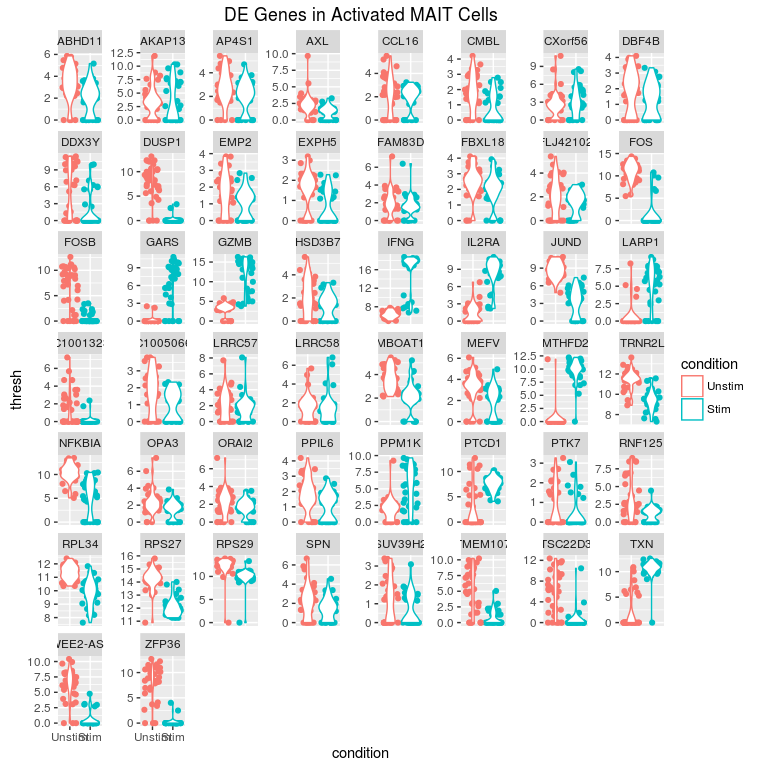Here is a standard violin plot showing how expression differs in each gene between stimulated and unstimulated cells. What could be improved about this visualization to make it more accurately reflect the model that’s being fit?

flat_dat[,lmPred:=lm(thresh~cngeneson + condition)\$fitted, key=symbolid]
ggbase +aes(x=cngeneson) + geom_line(aes(y=lmPred), lty=1) + xlab('Standardized Cellular Detection Rate')